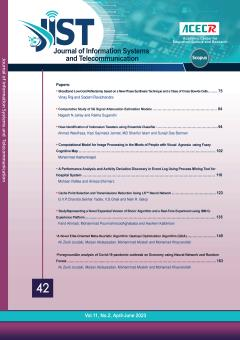• Home
• Seyed Alireza  Seyedin
• ### Current Issue### Browse

• #### List of ArticlesSeyed Alireza  Seyedin

• Open Access Article

1 - Security Analysis of Scalar Costa Scheme Against Known Message Attack in DCT-Domain Image Watermarking
This paper proposes an accurate information-theoretic security analysis of Scalar Costa Scheme (SCS) when the SCS is employed in the embedding layer of digital image watermarking. For this purpose, Discrete Cosine Transform (DCT) coefficients are extracted from the cove More
This paper proposes an accurate information-theoretic security analysis of Scalar Costa Scheme (SCS) when the SCS is employed in the embedding layer of digital image watermarking. For this purpose, Discrete Cosine Transform (DCT) coefficients are extracted from the cover images. Then, the SCS is used to embed watermarking messages into mid-frequency DCT coefficients. To prevent unauthorized embedding and/or decoding, the SCS codebook is randomized using the pseudorandom dither signal which plays the role of the secret key. A passive attacker applies Known Message Attack (KMA) on the watermarked messages to practically estimate the secret key. The security level is measured using residual entropy (equivocation) of the secret key provided that the attacker’s observations are available. It can be seen that the practical security level of the SCS depends on the host statistics which has contradiction with previous theoretical result. Furthermore, the practical security analysis of the SCS leads to the different values of the residual entropy in comparison with previous theoretical equation. It will be shown that these differences are mainly due to existence of uniform regions in images that cannot be captured by previous theoretical analysis. Another source of such differences is ignoring the dependencies between the observations of non-uniform regions in previous theoretical analysis. To provide an accurate reformulation, the theoretical equation for the uniform regions and the empirical equation for the non-uniform regions are proposed. Then, by combining these equations a new equation is presented for the whole image which considers both host statistics and observations dependencies. Finally, accuracy of the proposed formulations is examined through exhaustive simulations. Manuscript profile
• Open Access Article

2 - Active Steganalysis of Transform Domain Steganography Based on Sparse Component Analysis
Hamed Modaghegh Seyed Alireza  Seyedin
This paper presents a new active steganalysis method to break the transform domain steganography. Most of steganalysis techniques focus on detecting the presence or absence of a secret message in a cover (passive steganalysis), but in some cases we need to extract or es More
This paper presents a new active steganalysis method to break the transform domain steganography. Most of steganalysis techniques focus on detecting the presence or absence of a secret message in a cover (passive steganalysis), but in some cases we need to extract or estimate hidden message (active steganalysis). Although estimating the message is important but there is little research in this area. A new active steganalysis method which is based on Spars Component Analysis (SCA) technique is presented in this work. Here, the sparsity property of cover image and hidden message has been used to extract hidden message from stego image. In our method, transform domain steganography is formulated mathematically as a linear combination of sparse sources and therefore active steganalysis can be presented as a SCA problem. The feasibility of the SCA problem solving is confirmed by Linear Programming methods. Then, a fast algorithm is introduced to decrease the computational cost of steganalysis without much loss of accuracy. The accuracy of our new method has been confirmed in different experiments on a variety of transform domain steganography. These experiments show that, our method compared to the previous active steganalysis methods not only reduces the error rate but also decreases the computational cost. Manuscript profile
• Open Access Article

3 - Simultaneous Methods of Image Registration and Super-Resolution Using Analytical Combinational Jacobian Matrix
Hossein  Rezayi Seyed Alireza  Seyedin
In this paper we propose two new simultaneous image registration (IR) and super-resolution (SR) methods using a novel approach to calculate the Jacobian matrix. SR is the process of fusing several low resolution (LR) images to reconstruct a high resolution (HR) image; h More
In this paper we propose two new simultaneous image registration (IR) and super-resolution (SR) methods using a novel approach to calculate the Jacobian matrix. SR is the process of fusing several low resolution (LR) images to reconstruct a high resolution (HR) image; however as inverse problem it consists of three principal operations of warping, blurring and down-sampling should be applied to the desired HR image to produce the existing LR images. Unlike the previous methods, we neither calculate the Jacobian matrix numerically nor derive the Jacobian matrix by treating the three principal operations separately. We develop a new approach to derive the Jacobian matrix analytically from combinational form of the three principal operations. In this approach, a Gaussian kernel (as it is more realistic in a wide rang of applications) is considered for blurring, which can be adaptively resized for each LR image. The main intended method is established by applying the aforementioned ideas to the joint methods, a class of simultaneous iterative methods in which the incremental values for both registration parameters and HR image are obtained by solving one system of equations per iteration. Our second proposed method is formed by applying these ideas to the alternating minimization (AM) methods, a class of simultaneous iterative methods in which the incremental values of registration parameters are obtained after calculating the high resolution image at each iteration. The results show that our methods are superior to the recently proposed methods such as Tian's joint and Hardie's AM method. Additionally, the computational cost of our proposed methods has also been reduced. Manuscript profile